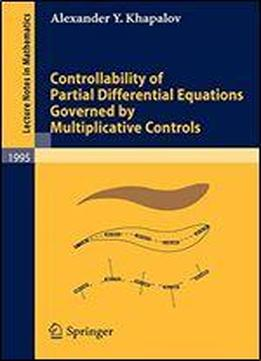# Controllability Of Partial Differential Equations Governed By Multiplicative Controls by Alexander Y. Khapalov / 2010 / English / PDF

This monograph addresses the global controllability of partial differential equations in the context of multiplicative (or bilinear) controls, which enter the model equations as coefficients. The methodology is illustrated with a variety of model equations.

views: 191